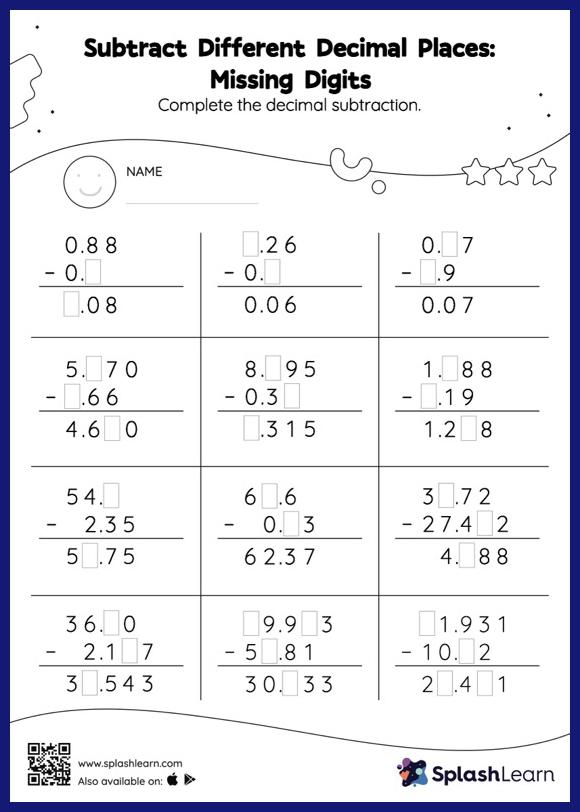# Subtract Different Decimal Places: Missing Digits Worksheet

Home > Subtract Different Decimal Places: Missing DigitsStudents demonstrate their problem-solving ability as they work on this subtract different decimal places worksheet. In this worksheet, students subtract decimals by aligning the decimal points and using zero as a placeholder. They then use the relationship between addition and subtraction to find the missing number in the subtract different decimal places worksheet. In each problem, the numbers are laid out in the vertical format. In this format, pair of digits in each successive place are tackled one by one. This helps students follow a structured approach.